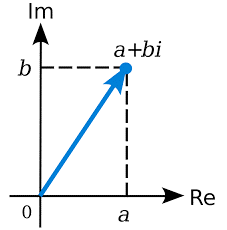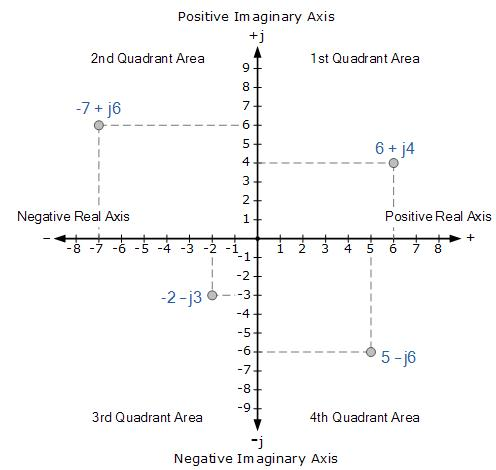Courses

# Revision Notes - Complex Numbers Mathematics Notes | EduRev

## Algebra for IIT JAM Mathematics

Created by: Veda Institute

## Mathematics : Revision Notes - Complex Numbers Mathematics Notes | EduRev

The document Revision Notes - Complex Numbers Mathematics Notes | EduRev is a part of the Mathematics Course Algebra for IIT JAM Mathematics.
All you need of Mathematics at this link: Mathematics

Definition of  Complex Number

Complex Numbers are the numbers which along with the real part also has the imaginary part included with it. That means complex numbers contains two different information included in it.
It is defined as the combination of real part and imaginary part. Either of the part can be zero.

 Complex Number Real Part Imaginary Part 3 + -5i 3 -5 5 5 0 -2i 0 -2

If ‘a’ is the real part and ‘b’ represents imaginary part, then complex number is represented as z = a + ib where i, stands for iota which itself is a square root of negative unity. Thus, we can also write z = Re(z) + i Im(z). This form of representation is also called as the Cartesian or algebraic Keep Calm and Love Complex Numberform of representation.
If z = -2 + j4, then Re(z) = -2 and Im(z) = 4.
Similarly, for z = 3+j5, Re(z) = 3 and Im(z) = (5).
Complex Number can be considered as the super-set of all the other different types of number. The set of all the complex numbers are generally represented by ‘C’.
Complex Numbers extends the concept of one dimensional real numbers to the two dimensional complex numbers in which two dimensions comes from real part and the imaginary part.

Few Examples of Complex Number:
2+i3,-5+6i,23i,(2-3i),(12-i1),3i are some of the examples of complex numbers. All the examples listed here are in Cartesian form. There are also different ways of representation for the complex number, which we shall learn in the next section.

Representation of Complex Number
Complex Number itself has many ways in which it can be expressed. Some of the most commonly used forms are:
• Cartesian or algebraic or rectangular form
• Trigonometric or polar form
• Exponential form
• Vector form

Can we take the square-root of a negative number?
Yes of course, but to understand this question, let’s go into more deep of complex numbers,
Consider the equation x2+1 = 0, If we try to get its solution, we would stuck at x = √(-1) so in Complex Number we assume that √(-1) =i or i2 =-1
which means i can be assumed as the solution of this equation. i is called as Iota in Complex Numbers.
We can further formulate as,
i2 = -1
i3 = i2*i=-i
i4 =i2*i2=1
So, we can say now, i4n = 1 where n is any positive interger.
Also, note that i + i2 + i3 + i4 = 0 or in + i2n + i3n + i4n= 0
This means sum of consecutive four powers of iota leads the result to zero.

What is the form a + ib?
We know from the above discussion that, Complex Numbers can be represented in four different ways. Out of which, algebraic or rectangular form is one of the form.
Z = a + ib is the algebraic form in which ‘a’ represents real part and ‘b’ represents imaginary part. Two mutually perpendicular axes are used to locate any complex point on the plane. Horizontal axis represents real part while the vertical axis represents imaginary part.How do we locate any Complex Number on the plane?
Let us take few examples to understand that, how can we locate any point on complex or argand plane?

Example 1:
Consider a complex number z = 6 +j4 (‘i’ and ‘j’, both can be used for representing imaginary part), if we compare this number with z = a + jb form. Then we can easily equate the two and get a = 6 and b = 4. Since both a and b are positive, which means number will be lying in the first quadrant. ‘z’ will be 6 units in the right and 4 units upwards from the origin. You can see the same point in the figure below.

Example 2:
Now consider a point in the second quadrant that is. z = -7 + j6, Here since a= -7 and b = 6 and thus will be lying in the second quadrant.
Point z is 7 units in the left and 6 units upwards from the origin. Refer the figure to understand it pictorially.

Example 3:
Now let’s consider a point in the third quadrant as z = -2 – j3. Since in third quadrant both a and b are negative and thus a = -2 and b = -3 in our example. This point will be lying 2 units in the left and 3 units downwards from the origin.

Example 4:
Let now take the fourth (of fourth quadrant) and the last case where z = 5 – j6. Here, a = 5 and b = - 6 i.e. a positive and b negative. This point will be lying 5 units in the right and 6 units downwards.What is i in math?
‘i’ (or ‘j’ in some books) in math is used to denote the imaginary part of any complex number. It helps us to clearly distinguish the real and imaginary part of any complex number. Moreover, i is just not to distinguish but also has got some value.
i = √(-1)
Main application of complex numbers is in the field of electronics. In electronics, already the letter ‘i’ is reserved for current and thus they started using ‘j’ in place of i for the imaginary part.

Are all Real Numbers are Complex Numbers?
Complex number has two parts, real part and the imaginary part.
that is. z = a + ib
if b = 0, z = a which is called as the Purely Real Number
and if a = 0, z = ib which is called as the Purely Imaginary Number.
Thus we can say that all real numbers are also complex number with imaginary part zero.

What is Complex Equation?
Any equation involving complex numbers in it are called as the complex equation.
For example: x = (2+3i) (3+4i), In this example, x is a multiple of two complex numbers. On multiplying these two complex number we can get the value of x.
z+ 2z + 3 = 0 is also an example of complex equation whose solution can be any complex number.

What is the application of Complex Numbers?
Complex Numbers have wide verity of applications in a variety of scientific and related areas such as electromagnetism, fluid dynamics, quantum mechanics, vibration analysis, cartography and control theory.
Let us have a look at the types of questions asked in the exam from this topic:
Illustration 1: Let a and b be roots of the equation x2 + x + 1 = 0. Then find the equation whose roots are a19 and b7.
Solution: Given that x2+x+1=0.
This means that either x =ω or x=ω2.
Hence, a19= ω19and b7 = ω142
Hence, the equation becomes x2–(ω+ω2)x + ωω2=0.
Hence the required equation is x2+x+1=0.

Illustration 2: Dividing f(z) by z - i, we obtain the remainder i and dividing it by z + i, we get remainder 1 + i. Find the remainder upon the division of f(z) by z+ 1.
Solution: z–i=0 gives z = i.
Remainder when f(z) is divided by (z–i) = f(i).
Similarly, the remainder when f(z) is divided by (z+ i) = f(- i)…..(1)
According to question f(i) = i
and f(-i) = 1 + i..... (2)
Since, z2+ 1 is a quadratic expression, therefore remainder when f(z) is divided by z2+1 will be in general a linear expression.
Let g(z) be the quotient and az + b the remainder when g(z) is divided by z2 + 1.
Then f(z) = g(z) (z2+1) + az+b ..... (3)
So, f(i) = g(i) (i2+1) + ai+b = ai+b .… (4)
and f(-i) = g(-i) (i2+1) – ai + b = -ai +b .… (5)
From (1) and (4), we have b + ai = i .… (6)
from (2) and (5) we have b – ai = 1 + i …. (7)
Solving (6) and (7), we have b = 1/2 + i and a = i/2.
Hence, the required remainder  = az + b = 1/2 iz + 1/2 + i.

Illustration 3: Find all complex numbers z for which arg [(3z-6-3i)/(2z-8-6i)] = π/4 and |z-3+4i| = 3.
Solution: We have
Since arg(a + ib) = π/4, so tan π/4 = b/a which gives a = b
So, 6x2+6y2–36x–24y+66 = 12x–12y-12
So,x2+y2–8x–2y+13 = 0 . …… (1)
Again, |z–3+i| = 3 gives |x+iy-3+i| = 3
So, (x-3)2 + (y+1)2 = 9
This yields x2+y2-6x+2y+1=0 …. (2)
Subtracting (2) form (1), we have
-2x–4y+12=0
This gives x=–2y+6 … (3)
Putting the value of x in (2), we get
(-2y+6)2 + y2 – 6 (-2y+6) + 2y + 1 = 0
So, 5y2 – 10 y +1 = 0
So, y = (-10 ± 4√5) /10
= 1 ± 2/√5
So, x = – 2y + 6 = 4 ∓ (- 4)/√5
so, z = x + iy = 4 ∓ 4/√5 + i (1 ± 2/√5)

Q.1. Any integral power of ‘i’ (iota) can be expressed as
(a) ± 1
(b) ± i
(c) ± 1 or ± i
(d) none of these
Ans. (c)

Q.2. If z is purely real negative complex number then
(a) amp (z) = π
(b) amp (z) = – π
(c) amp (z) = π/2
(d) amp (z) = π/4
Ans. (a)

Q.3. arg (z1z2) =
(a) arg(z1) + arg(z2) + 2π
(b) arg(z1) + arg(z2)
(c) arg(z1) . arg(z2)
(d) arg(z1) + arg(z2) + 2kπ, k ∈ I
Ans. (d)

Q.4. 1 + ωr + ω2r =
(a) 0
(b) 3
(c) 0 if r is not a multiple of 3 and 3 if r is a multiple of 3.
(d) 0
Ans: (c)

Q.5. If ω1 = ω2 are the complex slopes of two lines, then
(a) If ω1 = ω2 then the lines are parallel.
(b) If ω1 + ω2 = 0 then the lines are parallel.
(c) If ω1 = ω2 then the lines are not parallel.
(d) If ω1 = ω2 then the lines are perpendicular.
Ans. (a)

Offer running on EduRev: Apply code STAYHOME200 to get INR 200 off on our premium plan EduRev Infinity!

,

,

,

,

,

,

,

,

,

,

,

,

,

,

,

,

,

,

,

,

,

;